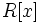# Normal ring

This article defines a property of commutative unital rings; a property that can be evaluated for a commutative unital ring
View all properties of commutative unital rings
VIEW RELATED: Commutative unital ring property implications | Commutative unital ring property non-implications |Commutative unital ring metaproperty satisfactions | Commutative unital ring metaproperty dissatisfactions | Commutative unital ring property satisfactions | Commutative unital ring property dissatisfactions

## Definition

A commutative unital ring is said to be normal if it is a reduced ring and further, if it is integrally closed in its total quotient ring.

This generalizes the notion of normal domain where we require the integral domain to be integrally closed in its field of fractions.

## Metaproperties

### Closure under taking localizations

This property of commutative unital rings is closed under taking localizations: the localization at a multiplicatively closed subset of a commutative unital ring with this property, also has this property. In particular, the localization at a prime ideal, and the localization at a maximal ideal, have the property.
View other localization-closed properties of commutative unital rings

The localization of a normal ring at any multiplicatively closed subset not containing zero, and hence, in particular, the localization relative to any prime ideal, is again a normal ring. For full proof, refer: Normality is localization-closed

### Closure under taking the polynomial ring

This property of commutative unital rings is polynomial-closed: it is closed under the operation of taking the polynomial ring. In other words, if$R$ is a commutative unital ring satisfying the property, so is$R[x]$

View other polynomial-closed properties of commutative unital rings

The polynomial ring over a normal ring is a normal ring. For full proof, refer: Normality is polynomial-closed